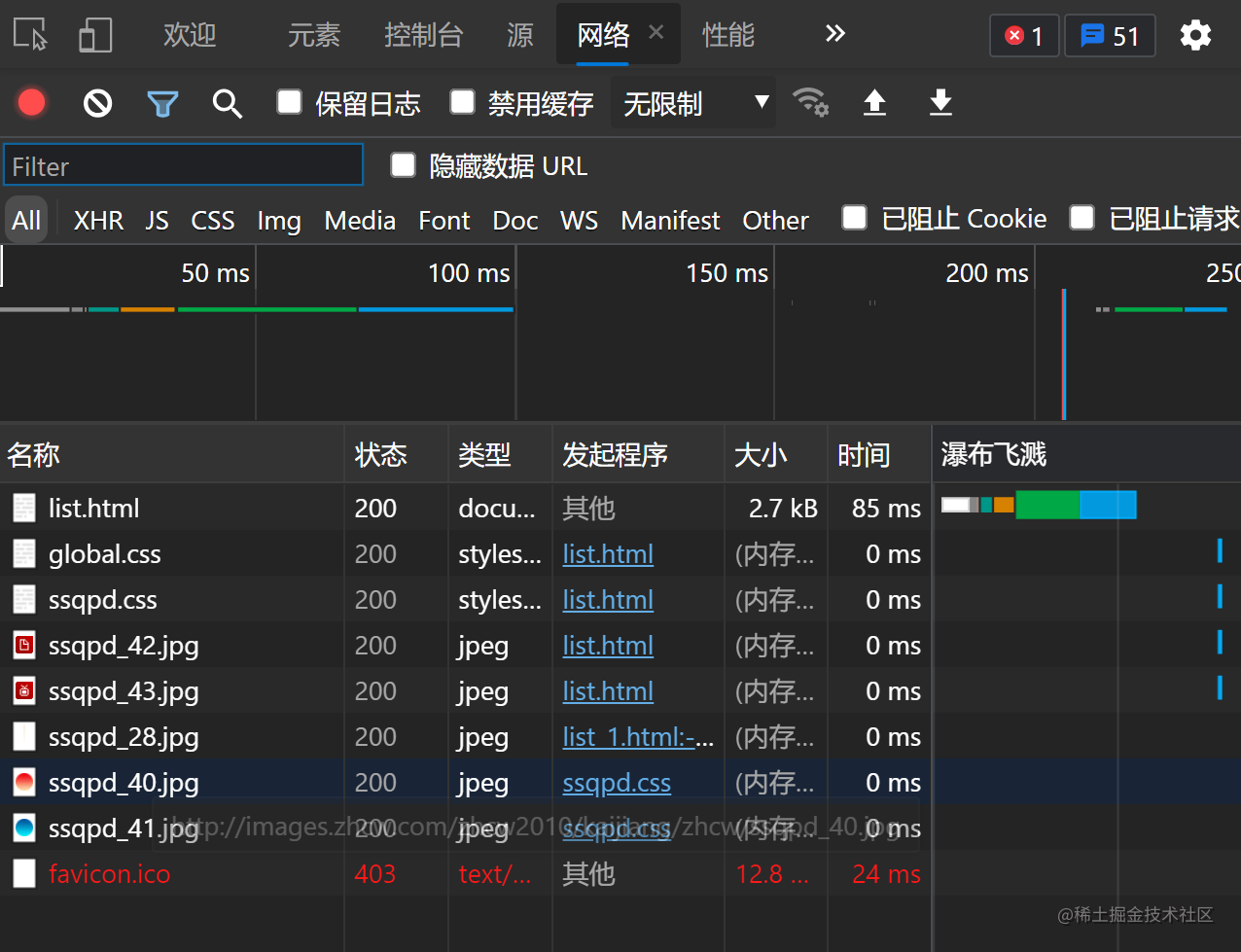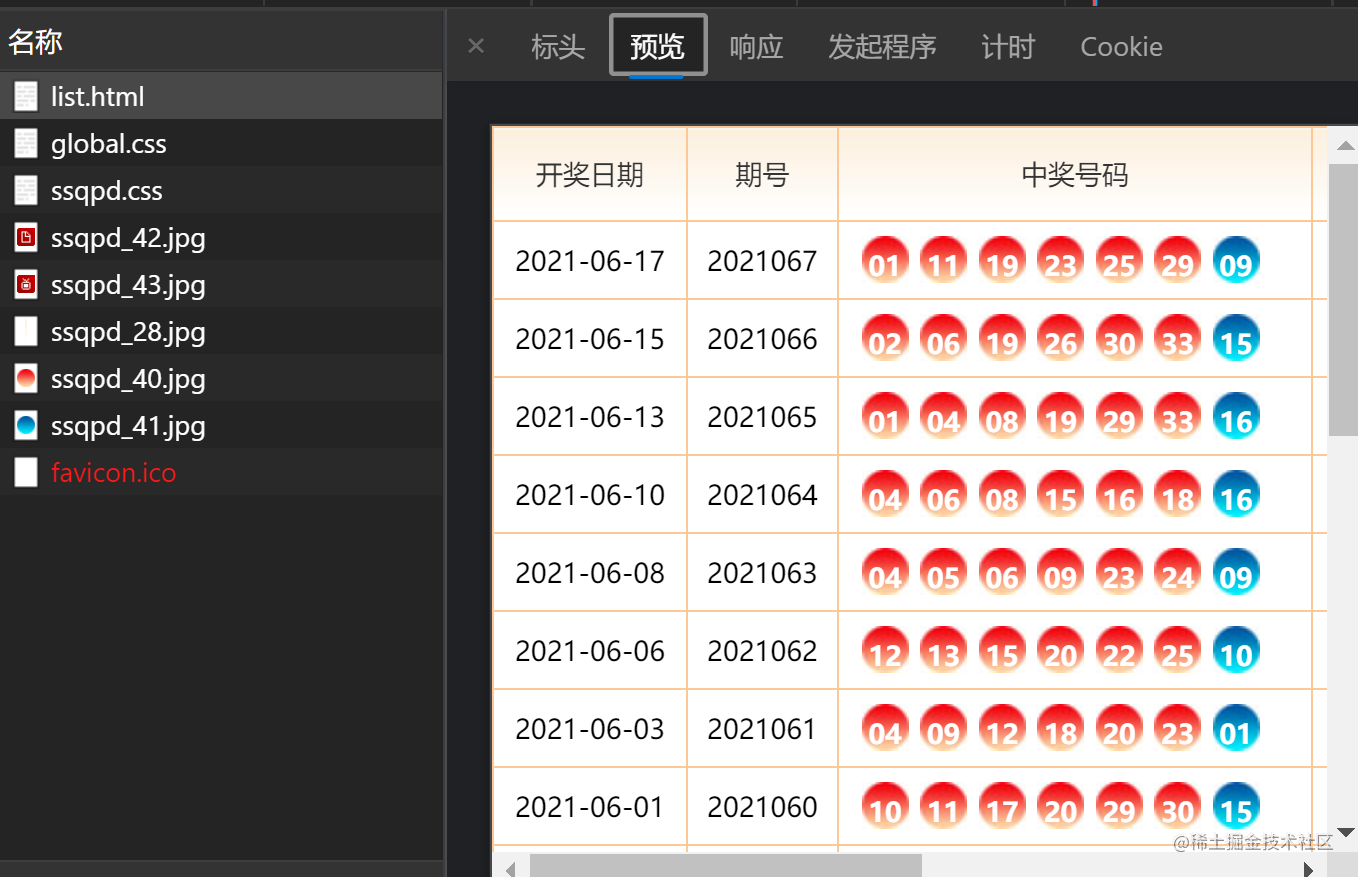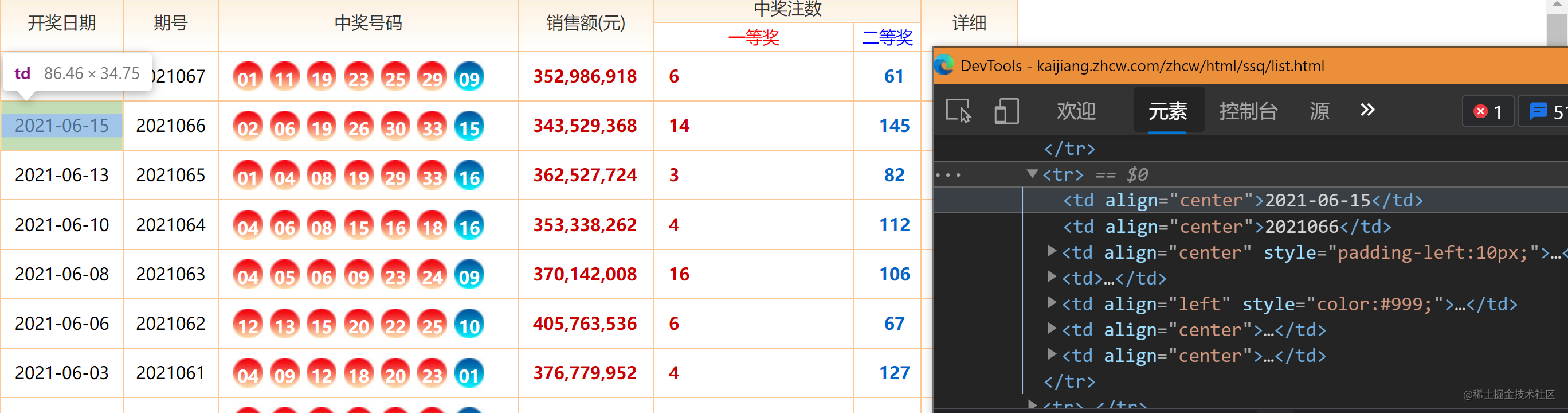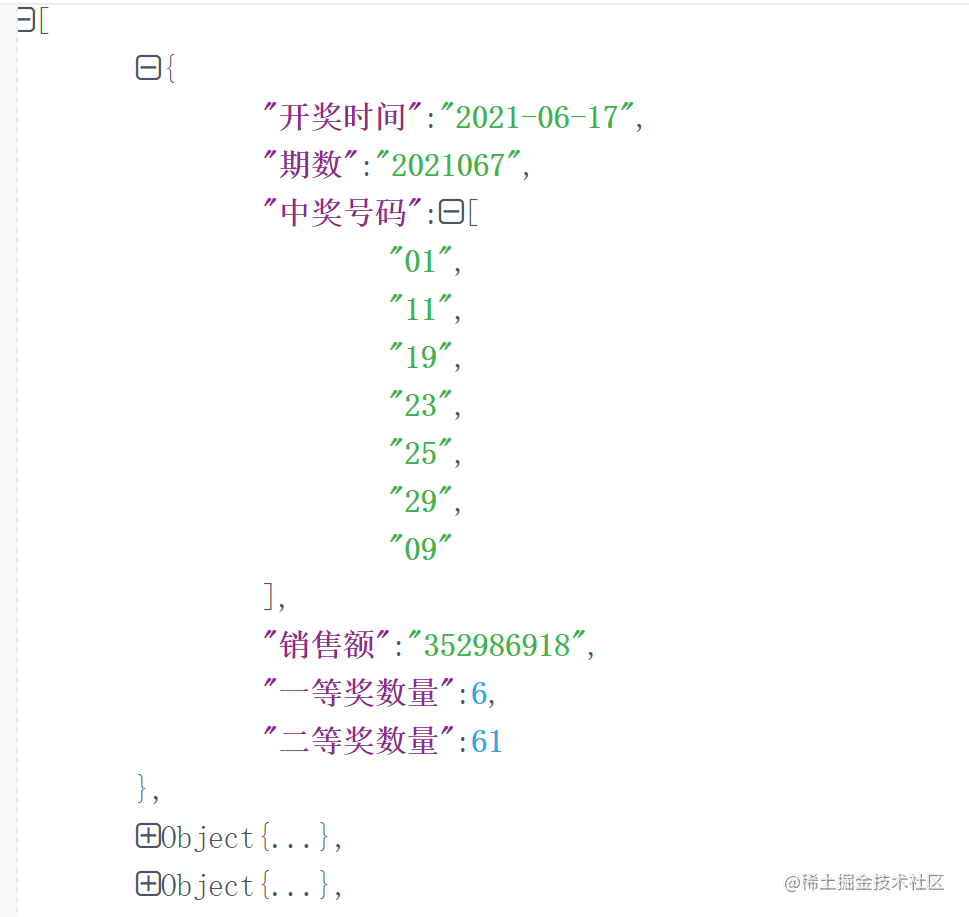# 使用deno爬取红蓝球号码

## 分析需求

1. 确定达成目标：定期获取红蓝球的号码。
2. 确定数据源：在这里
3. 确定从数据源获取数据的方法：分析网页结构1. 确定模拟请求和解析文档的工具
• 模拟请求：deno 实现了一套浏览器 api，可以使用 fetch 方法直接获取
• 解析文档：使用 deno-dom 库，可以将请求到的文档转换为 dom 树，这样就可以使用 `document.querySelectorAll` 这类方法来分析网页结构，获取数据。

## 实现

1. 获取文档
``````import { DOMParser, Element } from "deno-dom";

const result = await fetch(`http://kaijiang.zhcw.com/zhcw/html/ssq/list.html`);
const html = await result.text();

``````import { DOMParser, Element } from "deno-dom";

``````Relative import path "deno-dom" not prefixed with / or ./ or ../ from "file:///D:/caipiao/index.ts"

import_map 文件配置：

``````{
"imports": {
"deno-dom":"https://deno.land/x/deno_dom@v0.1.12-alpha/deno-dom-wasm.ts"
}
}

1. 分析文档内容``````<tr>
<!-- 开奖时间 -->
<td align="center">2021-06-15</td>
<!-- 期数 -->
<td align="center">2021066</td>
<!-- 红色球，class="rr" -->
<em class="rr">02</em>
<em class="rr">06</em>
<em class="rr">19</em>
<em class="rr">26</em>
<em class="rr">30</em>
<em class="rr">33</em>
<!-- 唯一的蓝球 -->
<em>15</em>
</td>
<!-- 销售额 -->
<td><strong>343,529,368</strong></td>
<!-- 一等奖数量 -->
<td align="left" style="color:#999;"><strong>14</strong></td>
<!-- 二等奖数量 -->
<td align="center"><strong class="rc">145</strong></td>
<td align="center">
<a href="http://www.zhcw.com/ssq/kjgg/" target="_blank"><img
src="http://images.zhcw.com/zhcw2010/kaijiang/zhcw/ssqpd_42.jpg" width="16" height="16"
align="absmiddle" title="详细信息"></a>
<a href="http://www.zhcw.com/video/kaijiangshipin/" target="_blank"><img
src="http://images.zhcw.com/zhcw2010/kaijiang/zhcw/ssqpd_43.jpg" width="16" height="16"
align="absmiddle" title="开奖视频"></a>
</td>
</tr>

• 每一组中奖数据按照开奖时间、期数、中奖号码、销售额、一等奖数量、二等奖数量依次排序
• 中奖号码中，蓝色球在最后一个位置。每个中奖号码均被 `<em>` 标签包裹

``````const doc = new DOMParser().parseFromString(html, "text/html");
if (doc) {
// 去掉表头。从第三行开始包含中奖数据，前两行一定是表头。
const rows = Array.from(doc.querySelectorAll("tr"))
const dataRows = rows.slice(2, rows.length - 1);
const data = dataRows.map((row) => {
const cells = Array.from((row as Element).querySelectorAll("td"));
const json = cells.reduce((collection, value, index) => {
// 每一组中奖数据按照开奖时间、期数、中奖号码、销售额、一等奖数量、二等奖数量依次排序
const dataKey = [
"开奖时间", "期数", "中奖号码", "销售额", "一等奖数量", "二等奖数量"
]

// 评估结果
const evalFunctions = [
(cell: Element) => cell.innerText,
(cell: Element) => cell.innerText,
(cell: Element) => Array.from(cell.querySelectorAll('em')).map(em => (em as Element).innerText),
(cell: Element) => cell.innerText.replaceAll(',', ''),
(cell: Element) => parseInt(cell.innerText.trim()),
(cell: Element) => parseInt(cell.innerText),
]

if(index < dataKey.length){
// 只有在 dataKey 中的值，才存储
collection.set(dataKey[index], evalFunctions[index](value as Element));
}

return collection;
}, new Map())

// Map 转换为 Object
let obj = Object.create(null);
for (let [k, v] of json) {
obj[k] = v;
}
return obj;
});
}

``````deno run --allow-net --import-map=import_map.json index.ts